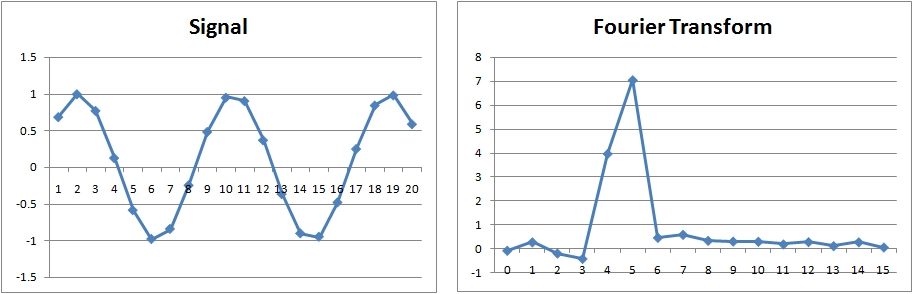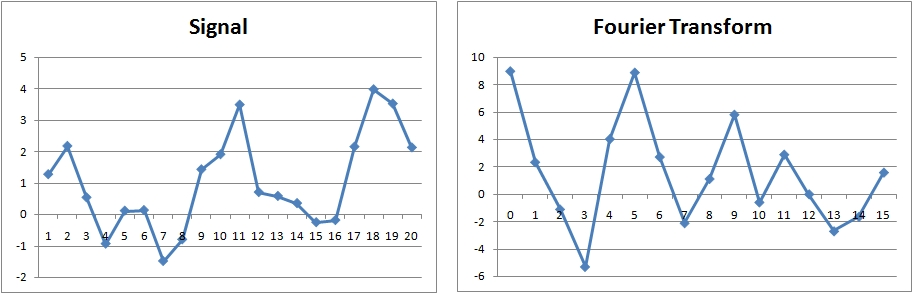# Fast Fourier Transform

The Fast Fourier Transform is one of the most used algorithms that are used to compute the Discrete Fourier Transform. The Fourier Transform is used in many applications given its ability to transform a time-series into its equivalent frequency representation.

The Fourier Transform X(k) of the time-series x(k)

is the following expression:

The Fourier Transform has several interesting properties:

• The first value of the transformed series X(0) is the average of the time-series (DC component).
• The highest frequency sample is called the Nyquist frequency. This is the maximum frequency that can be represented using a Fourier Transform. This frequency is also the maximum that must be present in the time-series in order for the signal to be reconstructed exactly from the transform.

Before applying the Fourier Transform to your data yu should cosider detrending it. Leaving a trend in place puts a lot of energy at the lowest frequencies and adds a DC component. This normally impaires the frequency estimate (see below). The Fourier Transform has several other interesting mathematical properties:

• Linearity: $a x_k + b y_k$ transforms into: $a X_k + b Y_k$
• Scaling: $x_{k \over a}$ transforms into: $a X_{a k}$
• Shifting: $x_{k+a}$ transforms into: $X_k e^{-2 j \pi a k}$
• Duality: ${1 \over N} x_{N-k}$ transforms into: $X_k$
• Convolution: $x_k * y_k$ transforms into: $X_k Y_k$

## Example Fourier Transforms

### Simple Sinusoidal### Simple Sinusoidal### Sinusoidal and Trend### Sinusoidal, Trend and NoiseReferences:

Cooley, James W., and John W. Tukey, 1965, “An algorithm for the machine calculation of complex Fourier series” H. V. Sorensen, D. L. Jones, M. T. Heideman, and C. S. Burrus, 1987, “Real-valued fast Fourier transform algorithms”

 Forecasting Methods Holt Winter’s, Series Decomposition and Wavelet Benchmarks Time Series Forecasting Use of the Moving Average in Time-series Forecasting Forecasting Concepts Denoising Techniques Error Statistics Computational Performance Fast Fourier Transform Moving Averages Kernel Smoothing Active Moving Average Savitsky-Golay Smoothing Fractal Projection Downloading Financial Data from Yahoo Multiple Regression Digital Signal Processing Principal Component Analysis Curve Analysis Options Pricing with Black-Scholes Markowitz Optimal Portfolio Time-series preprocessing